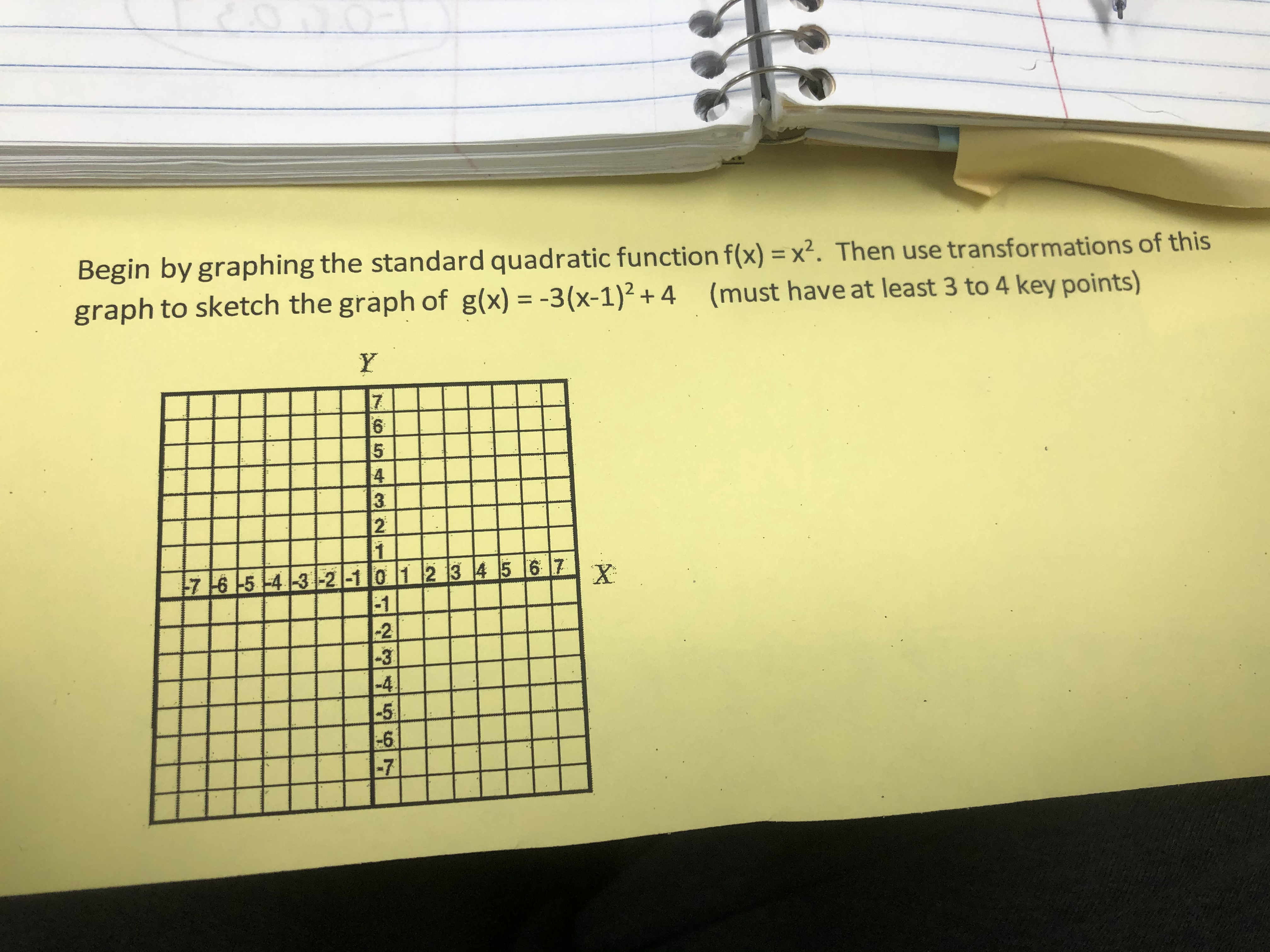# Begin by graphing the standard quadratic function f(x) = x2. Then use transformations of thisgraph to sketch the graph of g(x) = -3(x-1)2 + 4 (must have at least 3 to 4 key points)Y2217 16 -5 -4 3 -2 -10 1 2 3 45 6 7-1-2-3-4- 56-7X

Question
60 views

How do I solve this?help_outlineImage TranscriptioncloseBegin by graphing the standard quadratic function f(x) = x2. Then use transformations of this graph to sketch the graph of g(x) = -3(x-1)2 + 4 (must have at least 3 to 4 key points) Y 22 1 7 16 -5 -4 3 -2 -10 1 2 3 45 6 7 -1 -2 -3 -4 - 5 6 -7 X fullscreen
check_circle

Step 1

Sketch the graph of g(x)= -3(x-1)2+4, use the transformations of the graph and graphing the function f(x)=x2

Step 2

Graphical transformation

• f(x) transform to af(x), stretch the graph of f(x) ‘a’ time along y-axis, │a│>0

Step 3
• f(x) transform to f(x-a), a is positive shift the graph of f(...

### Want to see the full answer?

See Solution

#### Want to see this answer and more?

Solutions are written by subject experts who are available 24/7. Questions are typically answered within 1 hour.*

See Solution
*Response times may vary by subject and question.
Tagged in

### Algebra